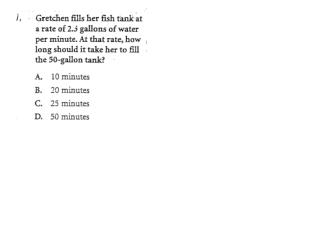DownloadDownload PresentationStandard 1 – Number and Numerical Operations

# Standard 1 – Number and Numerical Operations

Download Presentation## Standard 1 – Number and Numerical Operations

- - - - - - - - - - - - - - - - - - - - - - - - - - - E N D - - - - - - - - - - - - - - - - - - - - - - - - - - -
##### Presentation Transcript

1. Standard 1 – Number and Numerical Operations • The following are two rational numbers greater than 1 and less than 2: • ___ • 7/6, 1.234 • Give two more rational numbers greater than 1 and less than 2. Give reasons why your numbers are rational numbers. • The following are two irrational numbers greater than 1 and less than 2: π/2, 1.01001000100001… • Give two more irrational numbers greater than 1 and less than 2. Give reasons why your numbers are irrational numbers.

2. Plot the points on the grid provided: A(2,8), B(4,5), C(4,0), D(2,-3), E(-3,-3), F(-5,0), G(-5,5), H(-3,8). Draw figure ABCDEFGH. Find the Perimeter. Find the area. Show your work.

3. The counting numbers can be arranged in a table like the one given below. • Complete the 4th, 5th, and 6th rowa in the table. • What numbers are in the 100th row? • Write an expression for the Nth row of column D. Column C? Column E? • In which row and column will the number 59 be found? Expalin your answer. • In which row and column will the number 301 be found? Expalin your answer.